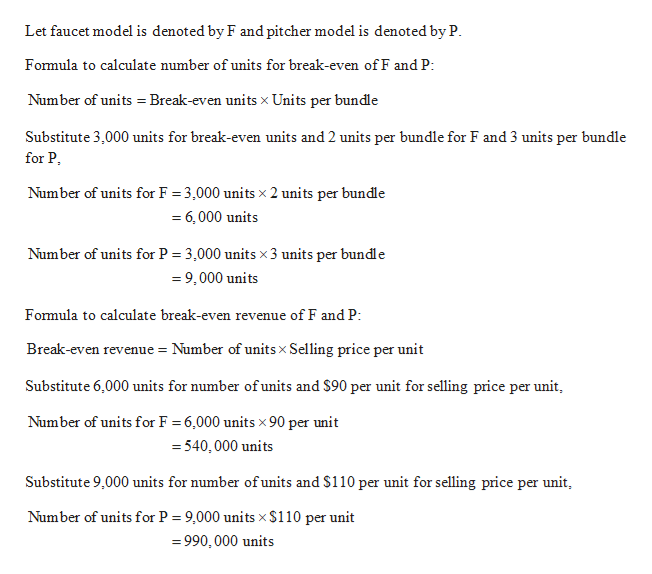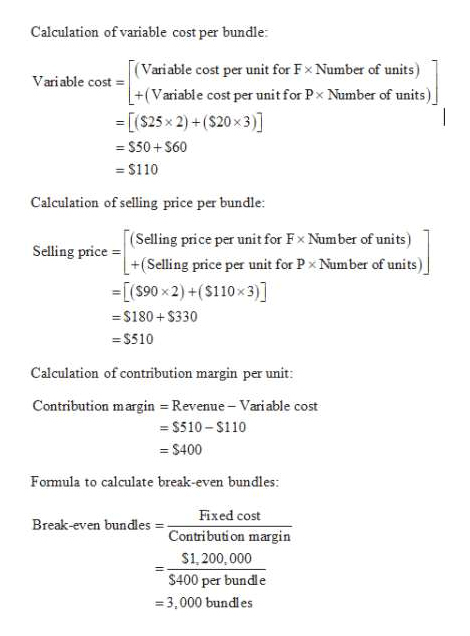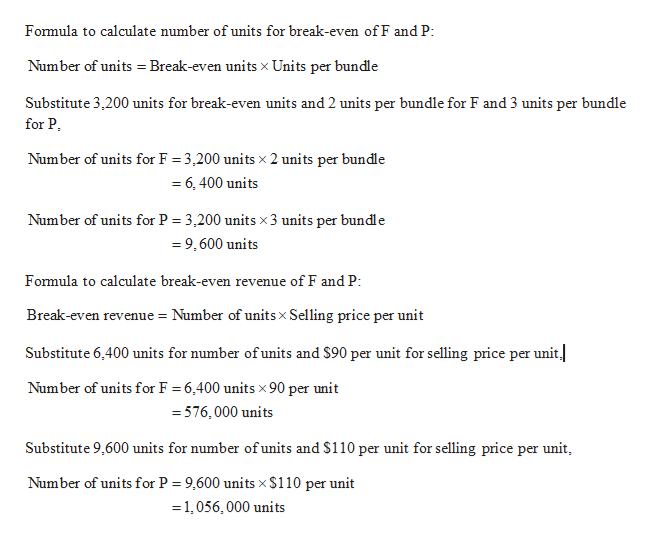# Multiproduct CVP and decision making. Crystal Clear Products produces two types of water filters. One attaches to the faucet and cleans all water that passes through the faucet. The other is a pitcher cum filter that only purifies water meant for drinking.  The unit that attaches to the faucet is sold for \$90 and has variable costs of \$25. The pitcher-cum-filter sells for \$110 and has variable costs of \$20. Crystal Clear sells two faucet models for every three pitchers sold. Fixed costs equal \$1,200,000.Required:What is the breakeven point in unit sales and dollars for each type of filter at the current sales mix?Crystal Clear is considering buying new production equipment. The new equipment will increase fixed cost by \$208,000 per year and will decrease the variable cost of the faucet and the pitcher units by \$5 and \$10, respectively. Assuming the same sales mix, how many of each type of filter does Crystal Clear need to sell to break even?Assuming the same sales mix, at what total sales level would Crystal Clear be indifferent between using the old equipment and buying the new production equipment? If total sales are expected to be 24,000 units, should Crystal Clear buy the new production equipment?

Question
20 views

Multiproduct CVP and decision making. Crystal Clear Products produces two types of water filters. One attaches to the faucet and cleans all water that passes through the faucet. The other is a pitcher cum filter that only purifies water meant for drinking.  The unit that attaches to the faucet is sold for \$90 and has variable costs of \$25. The pitcher-cum-filter sells for \$110 and has variable costs of \$20. Crystal Clear sells two faucet models for every three pitchers sold. Fixed costs equal \$1,200,000.

Required:

1. What is the breakeven point in unit sales and dollars for each type of filter at the current sales mix?
2. Crystal Clear is considering buying new production equipment. The new equipment will increase fixed cost by \$208,000 per year and will decrease the variable cost of the faucet and the pitcher units by \$5 and \$10, respectively. Assuming the same sales mix, how many of each type of filter does Crystal Clear need to sell to break even?
3. Assuming the same sales mix, at what total sales level would Crystal Clear be indifferent between using the old equipment and buying the new production equipment? If total sales are expected to be 24,000 units, should Crystal Clear buy the new production equipment?
check_circle

Step 1

1.

Compute Break-even units and break-even revenue for faucet model and pitcher model.help_outlineImage TranscriptioncloseLet faucet model is denoted by F and pitcher model is denoted by P. Formula to calculate number of units for break-even of F and P: Number of units = Break-even units x Units per bundle Substitute 3,000 units for break-even units and 2 units per bundle for F and 3 units per bundle for P, Number of units for F = 3,000 units x 2 units per bundle = 6, 000 units %3D Number of units for P = 3,000 units x 3 units per bundle = 9,000 units Formula to calculate break-even revenue of F and P: Break-even revenue = Number of units x Selling price per unit Substitute 6,000 units for number of units and \$90 per unit for selling price per unit, Number of units for F = 6,000 units x90 per unit = 540,000 units Substitute 9,000 units for number of units and \$110 per unit for selling price per unit, Number of units for P = 9,000 units x \$110 per unit = 990, 000 units fullscreen
Step 2

Working Note:help_outlineImage TranscriptioncloseCalculation of variable cost per bundle: [(Vari able cost per unit for Fx Number of units) +(Variable cost per unit for Px Number of units) = [(\$25 x 2) + (\$20 x3)] Variable cost = = \$50+ S60 = \$110 Calculation of selling price per bundle: (Selling price per unit for Fx Number of units) +(Selling price per unit for P x Number of units) =[(S90 x 2) +(\$110x3)] Selling price = = \$180 + \$330 = S510 Calculation of contribution margin per unit: Contribution margin = Revenue - Variable cost = S510 – S110 = \$400 Formula to calculate break-even bundles: Fixed cost Break-even bundles Contribution margin S1, 200,000 \$400 per bundle = 3,000 bundles fullscreen
Step 3

2.

Compute Break-even point in units and dol...help_outlineImage TranscriptioncloseFormula to calculate number of units for break-even of F and P: Number of units = Break-even units x Units per bundle Substitute 3,200 units for break-even units and 2 units per bundle for F and 3 units per bundle for P, Number of units for F = 3,200 units x 2 units per bundle = 6, 400 units Number of units for P = 3,200 units x 3 units per bundle = 9, 600 units Formula to calculate break-even revenue of F and P: Break-even revenue = Number of units x Selling price per unit Substitute 6,400 units for number of units and \$90 per unit for selling price per unit. Number of units for F = 6,400 units x 90 per unit = 576,000 units Substitute 9,600 units for number of units and \$110 per unit for selling price per unit, Number of units for P = 9,600 units x \$110 per unit = 1,056, 000 units fullscreen

### Want to see the full answer?

See Solution

#### Want to see this answer and more?

Solutions are written by subject experts who are available 24/7. Questions are typically answered within 1 hour.*

See Solution
*Response times may vary by subject and question.
Tagged in